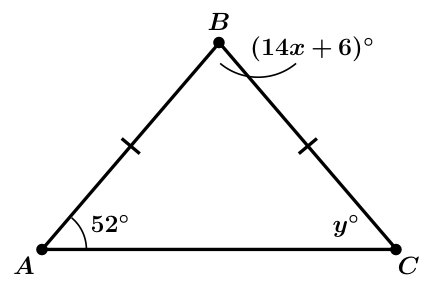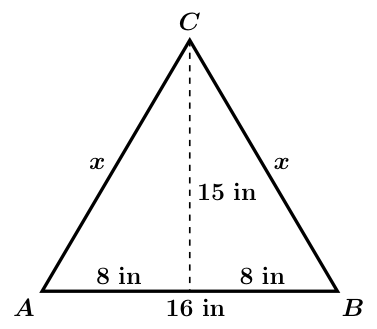# GEOM 2 | Lesson 2 | PracticeComplete the following problems to summarize the lesson:

1) What is the measure of $$\angle{C}$$?2) What is the measure of $$\angle{B}$$?3) Calculate the value of t.4) Calculate the value of x.5) Are the 2 triangles right, acute, or obtuse?Check solutions here.